# i need the answer quickly fast as you can Q3: Determine the vertical and horizontal displacement...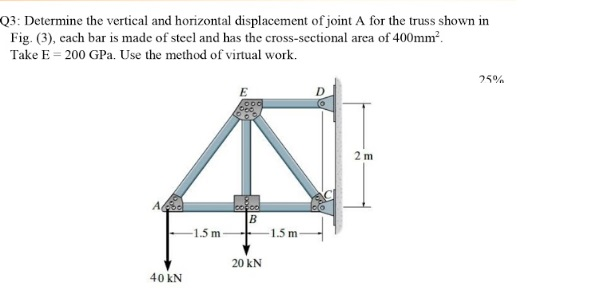i need the answer quickly fast as you can

Q3: Determine the vertical and horizontal displacement of joint A for the truss shown in Fig. (3), cach bar is made of steel and has the cross-sectional area of 400mm? Take E = 200 GPa. Use the method of virtual work. 25% E 2 m А. cool B 1.5 m -1.5 m 20 KN 40 KN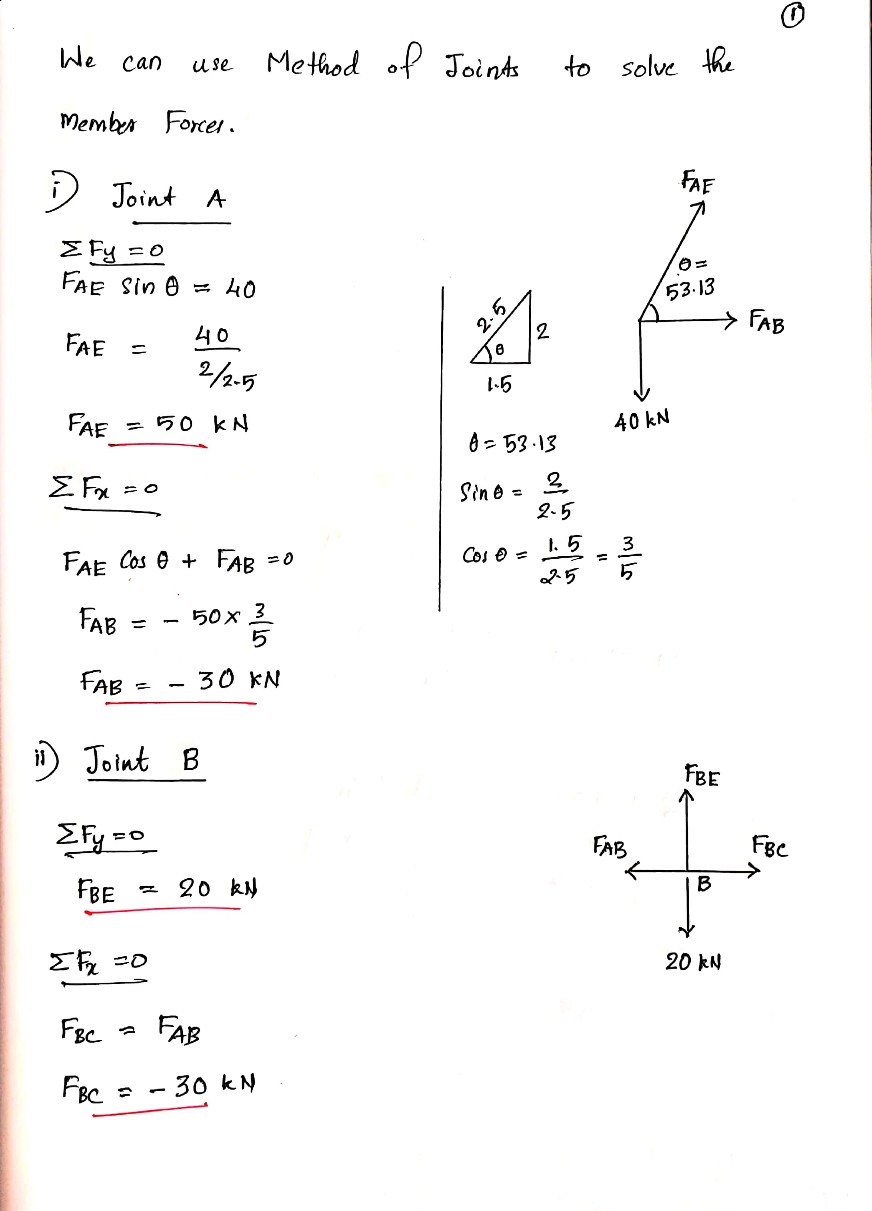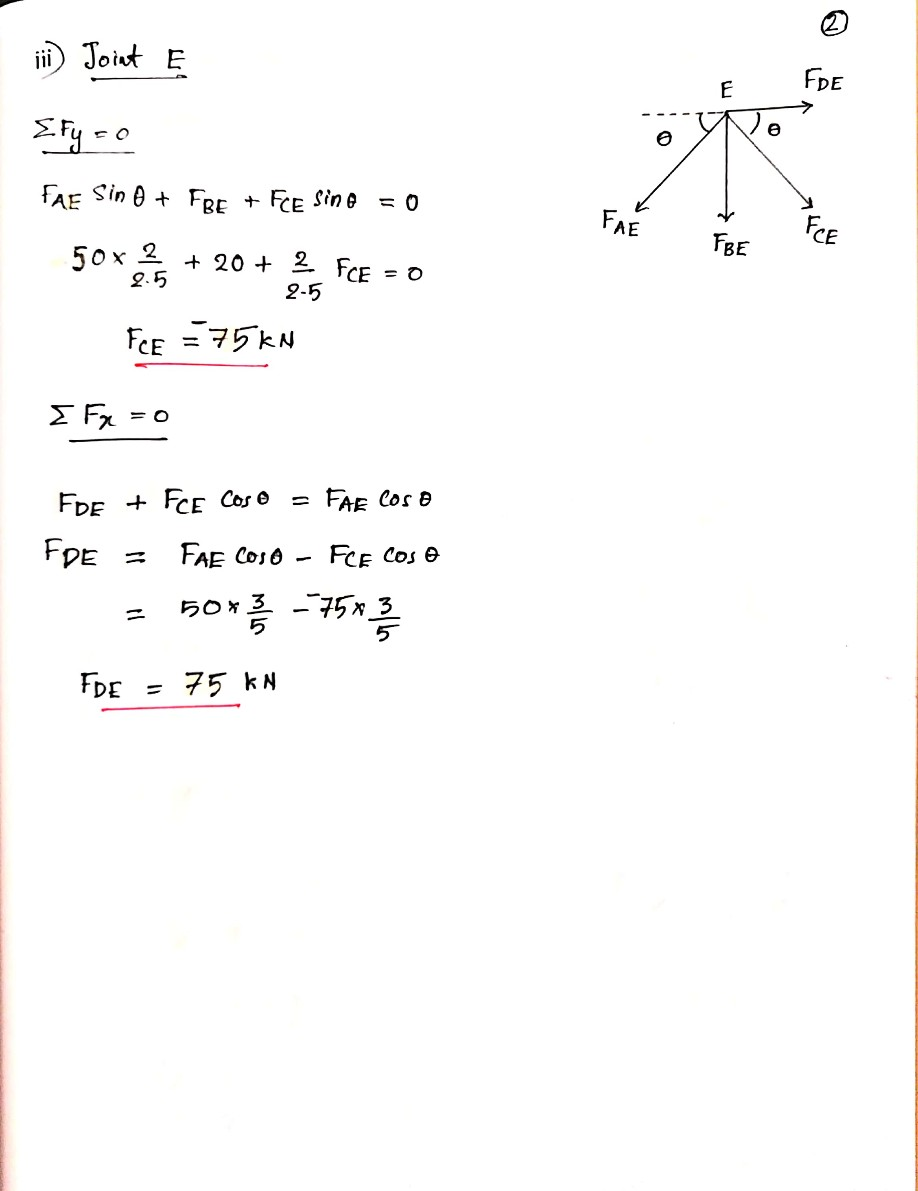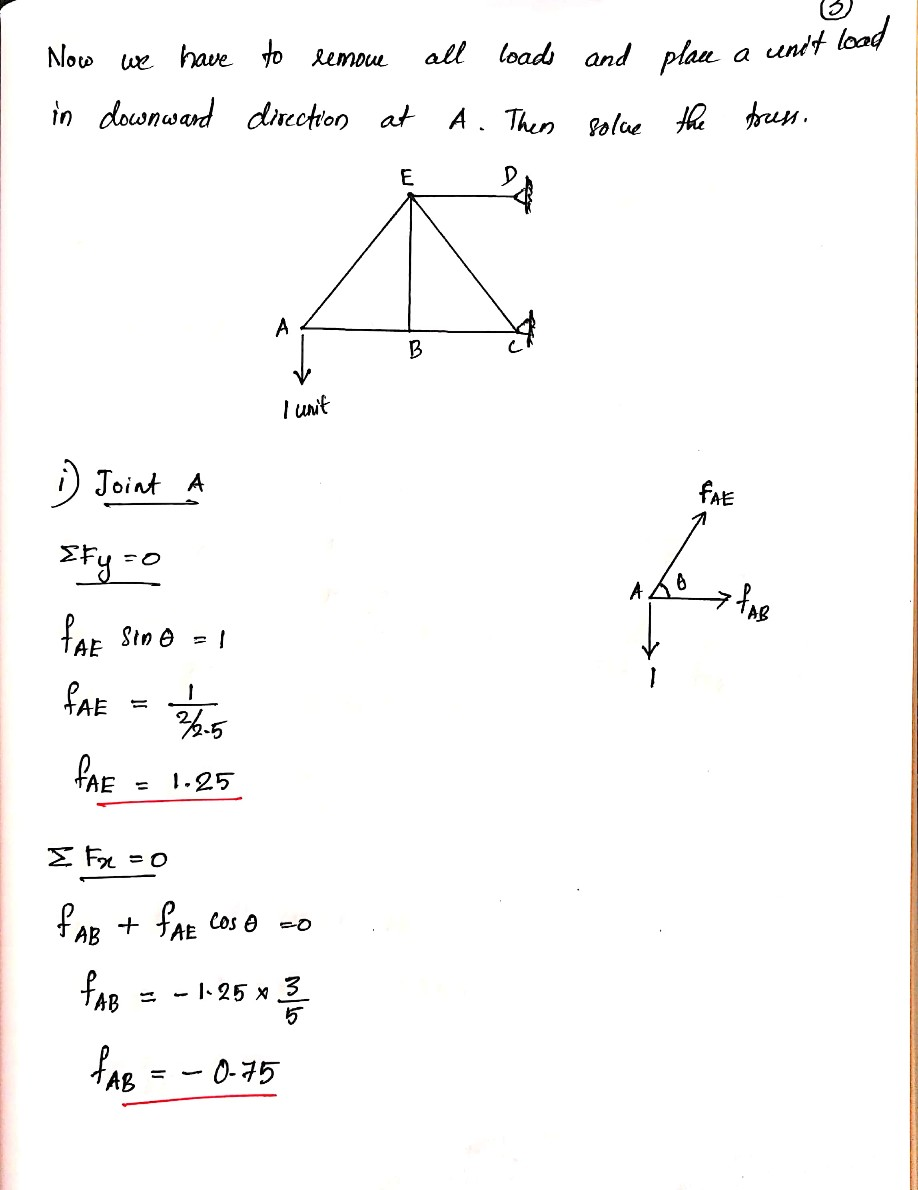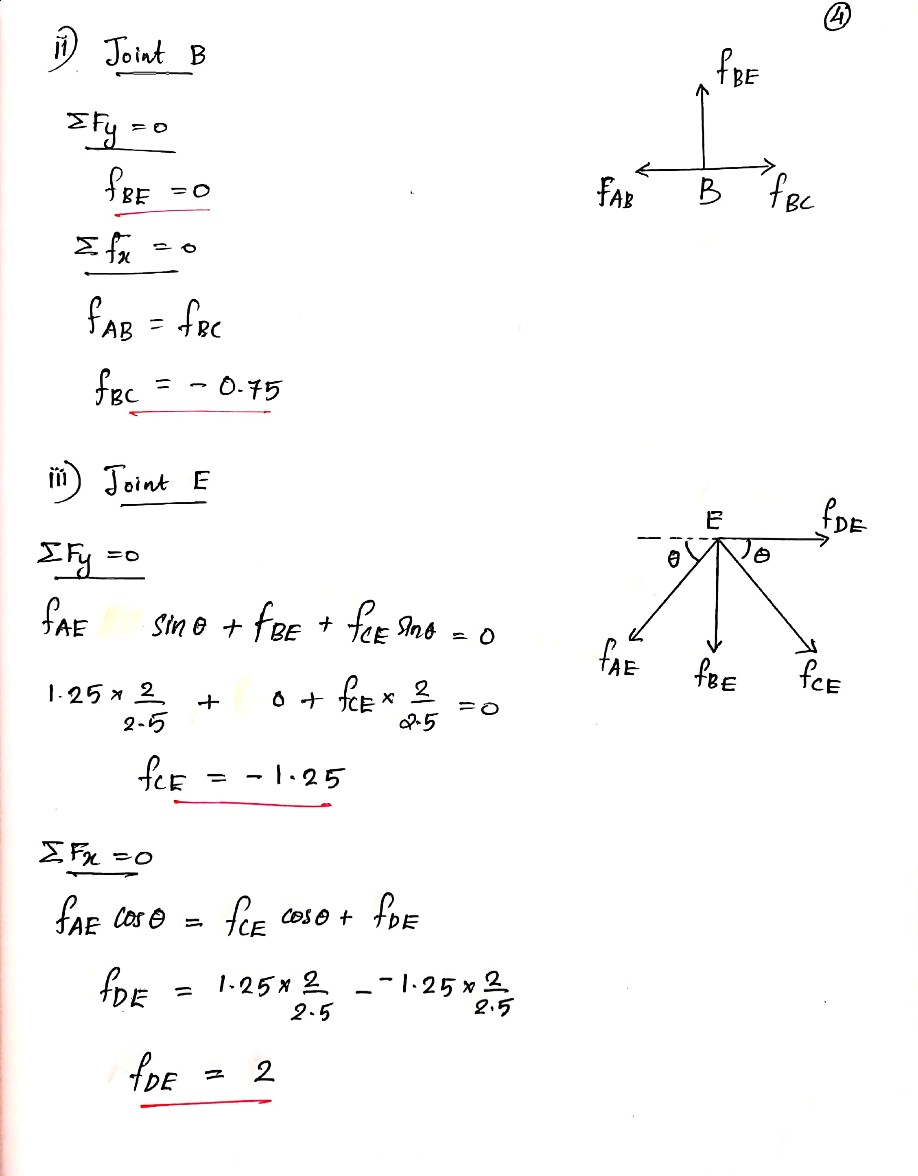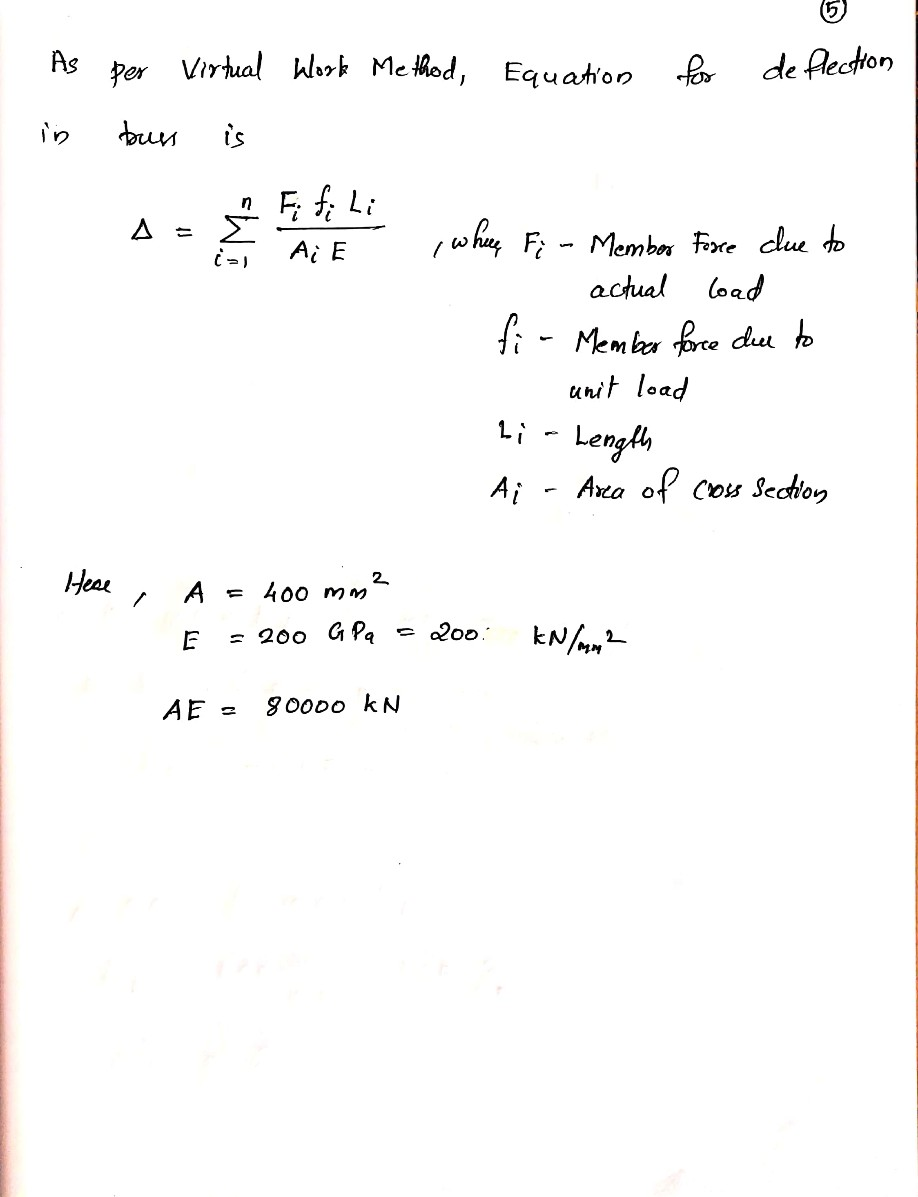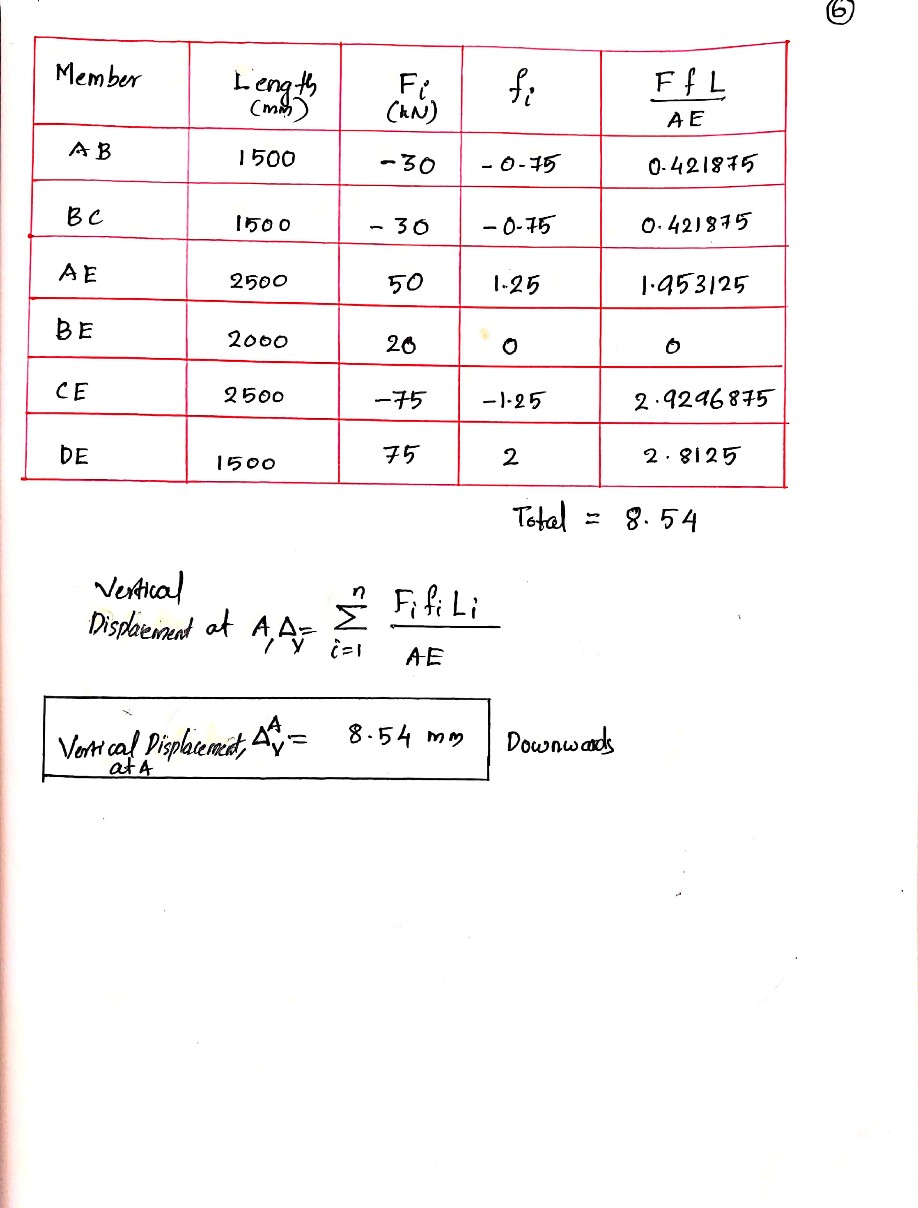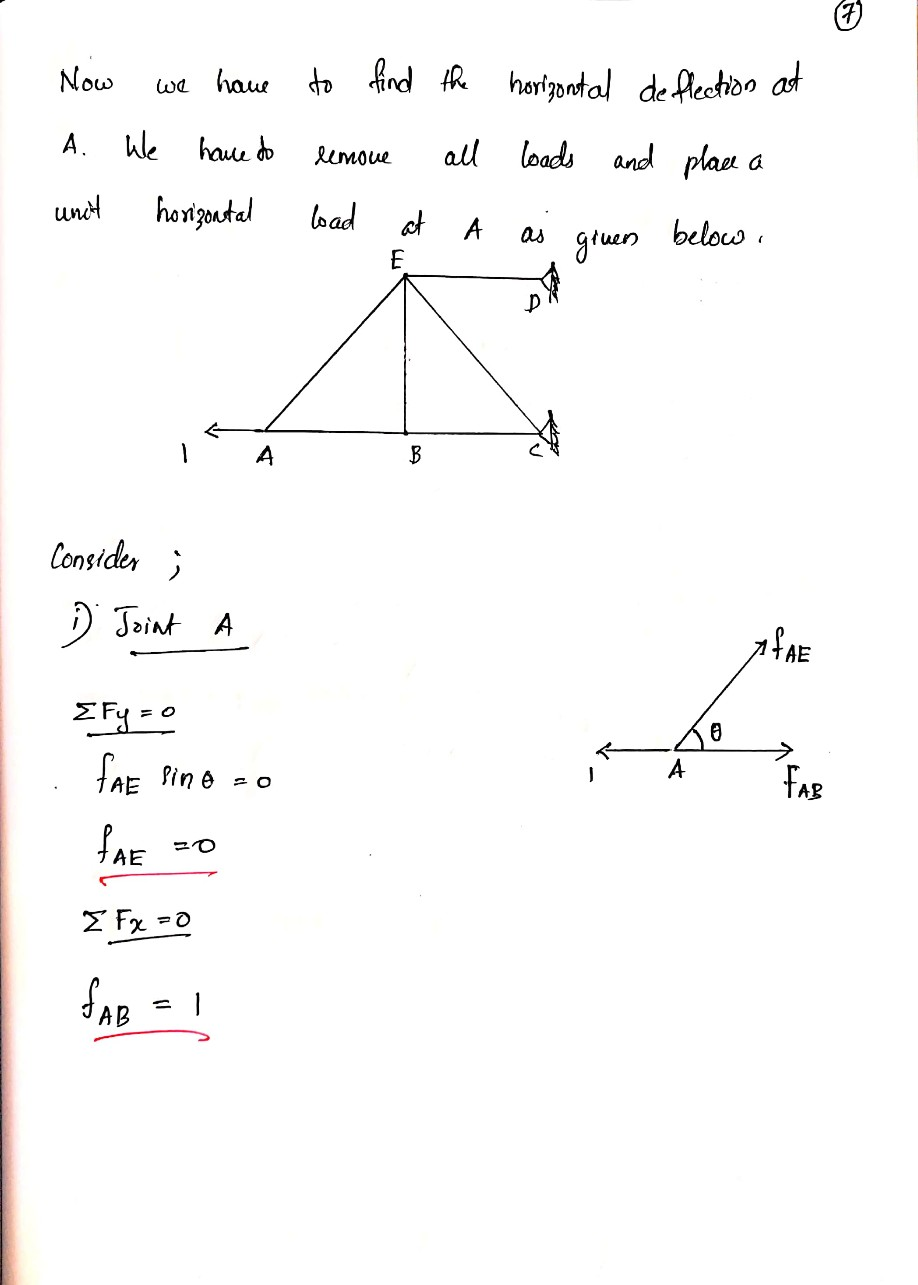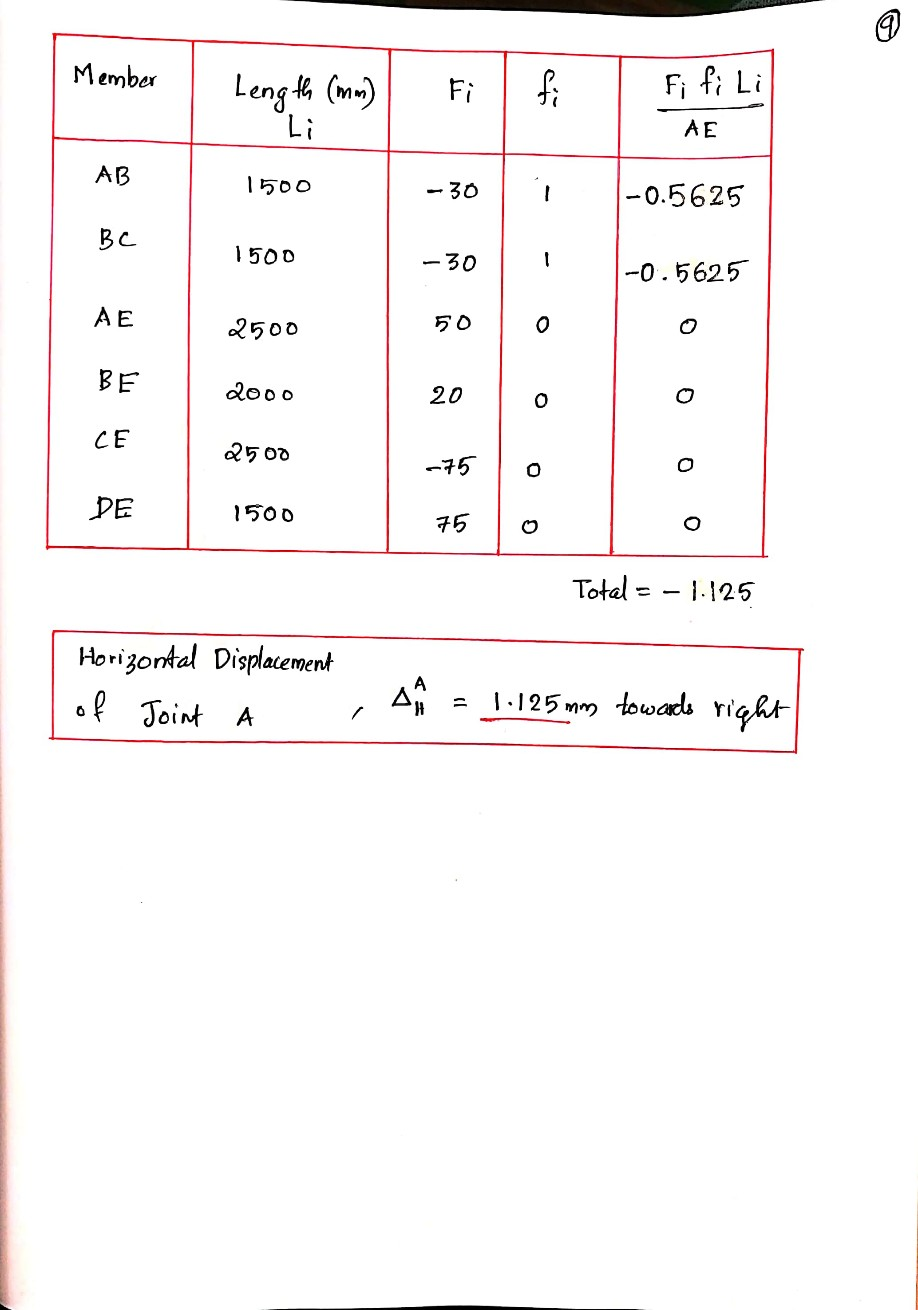The given truss is solved using method of joints and the Displacement of joint A is found using Virtual Work Method.

#### Earn Coin

Coins can be redeemed for fabulous gifts.

Similar Homework Help Questions
• ### Q3: Determine the vertical and horizontal displacement of joint A for the truss shown in Fig....Q3: Determine the vertical and horizontal displacement of joint A for the truss shown in Fig. (3). each bar is made of steel and has the cross-sectional area of 400mm Take E = 200 GPa Use the method of virtual work. E D 2 m |в -1.5 m -1.5 m 20 KN 40 KN Fig. (3)

• ### Solve It fast Please Q3: Determine the vertical and horizontal displacement of joint A for the...Solve It fast Please Q3: Determine the vertical and horizontal displacement of joint A for the truss shown in Fig. (3). cach bar is made of steel and has the cross-sectional area of 400mmº. Take E = 200 GP. Use the method of virtual work. 25% E OO D 2 m AAS ered B -1.5 m -1.5 m 20 KN 40 KN Fig. (3)

• ### Q3: Determine the vertical and horizontal displacement of joint A for the truss shown in Fig....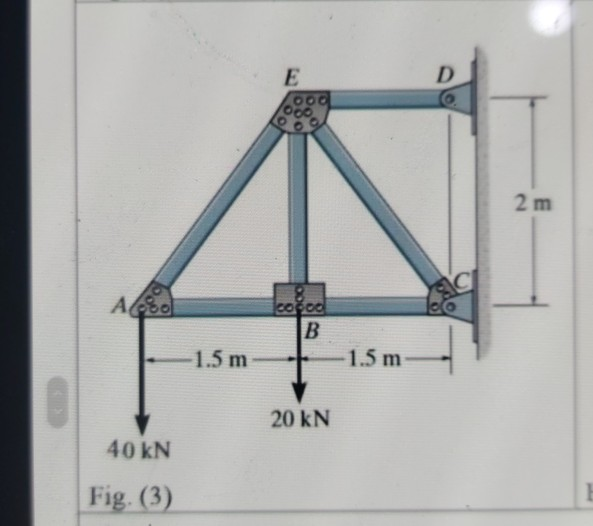Q3: Determine the vertical and horizontal displacement of joint A for the truss shown in Fig. (3), each bar is made of steel and has the cross-sectional area of 400mm?. Take E = 200 GPa. Use the method of virtual work. E D 00. 2 m СІ Ao cocoa B 1.5 m 1.5 m 20 KN 40 kN Fig. (3)

• ### stion 1 Determine the total vertical displacement of joint A (due to the load and tempreature...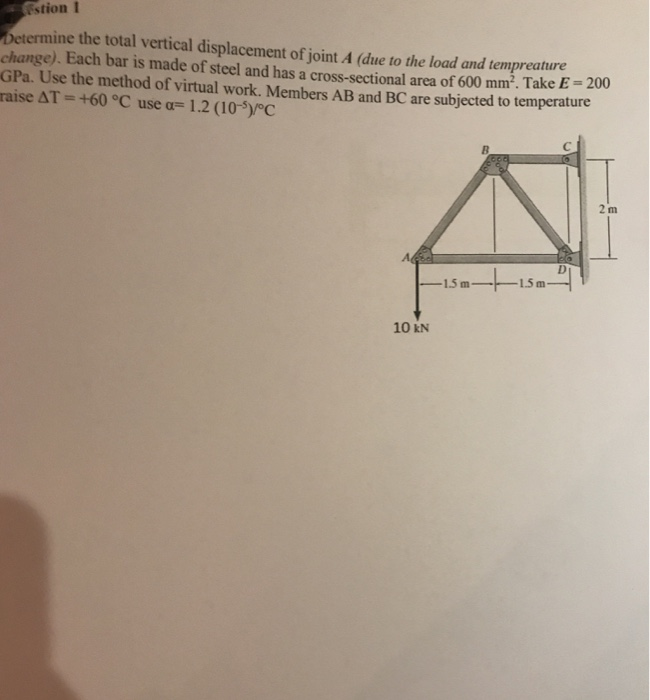stion 1 Determine the total vertical displacement of joint A (due to the load and tempreature change). Each bar is made of steel and has a cross-sectional area of 600 mm². Take E - 2001 GPa. Use the method of virtual work. Members AB and BC are subjected to temperature raise AT = +60 °C use a= 1.2 (10-5)/°C —15 m- 1.5m 10 KN

• ### Please show all work 4 kN 2. Use Castigliano's theorem to find the vertical displacement of...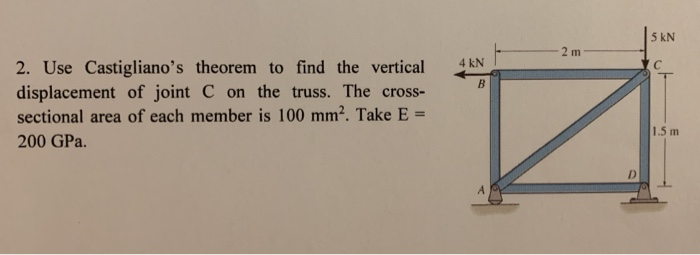Please show all work 4 kN 2. Use Castigliano's theorem to find the vertical displacement of joint C on the truss. The cross- sectional area of each member is 100 mm2. Take E = 200 GPa. 1.5 m

• ### Use the method of Virtual Work and compute the vertical and horizontal displacement of joint B of the truss. Each steel member has a cross-sectional area of 300mm, E=200GPa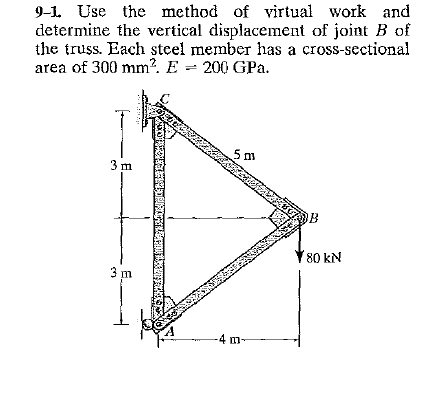Use the method of Virtual Work and compute the vertical and horizontal displacement of joint B of the truss. Each steel member has a cross-sectional area of 300mm^2. E=200GPa

• ### 15 m B- Question 3: Each member of the truss shown is made of steel (E- 2 1 0 GPa ) and has a cro...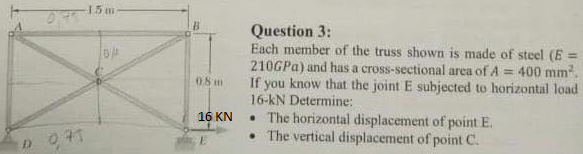15 m B- Question 3: Each member of the truss shown is made of steel (E- 2 1 0 GPa ) and has a cross-sectional area of A If you know that the joint E subjected to horizontal load 16-kN Determine: .The horizontal displacement of point E . The vertical displacement of point C. 400 mm2 0.8m 16 KN 15 m B- Question 3: Each member of the truss shown is made of steel (E- 2 1 0 GPa )...

• ### 14–81. Determine the horizontal displacement of jou on the truss. Each A992 steel member has a...14–81. Determine the horizontal displacement of jou on the truss. Each A992 steel member has a cross-sect area of 1935 mm. Splacement of joint C 14-82. Determine the horizontal displacement of joint B on the truss. Each A992 steel member has a cross-sectional area of 1935 mm. 10 KN C 75 kN 1.2 m obdo be255 zil bado Todo IA a D - 0.9 m- Probs. 14-81/82

• ### oblem 2 etermi ine the vertical displacement at joint A. Each bar is made of eel...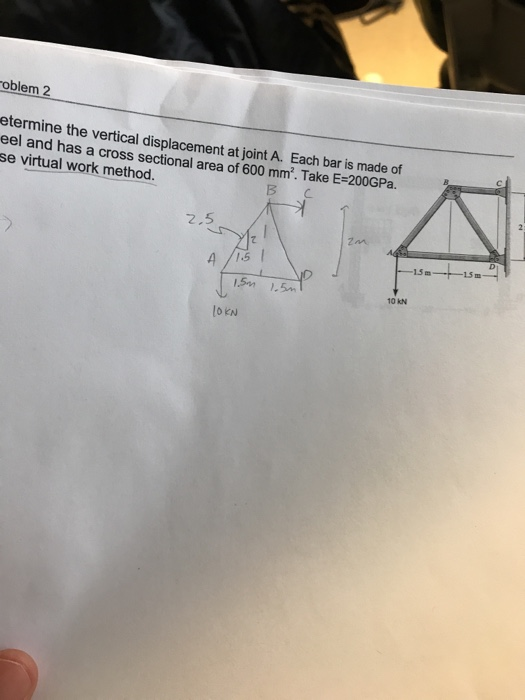oblem 2 etermi ine the vertical displacement at joint A. Each bar is made of eel and has a cross sectional area of 600 mm. Take E-200GPa. se virtual work method. ВС 2.5 15m 15 m 10 kN OKN

• ### A-2 Determine the vertical displacement of joint 1. Each bar is made of steel and has...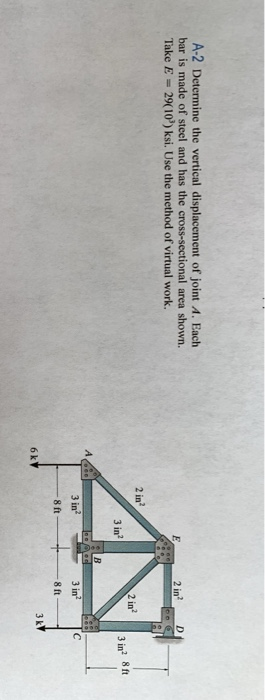A-2 Determine the vertical displacement of joint 1. Each bar is made of steel and has the cross-sectional area shown. Take E = 29(10) ksi. Use the method of virtual work. E 2 in? D 2 in? 2 in? 3 in? 3 in 8 ft 3 in с 8 ft 8 ft 3kV 6k